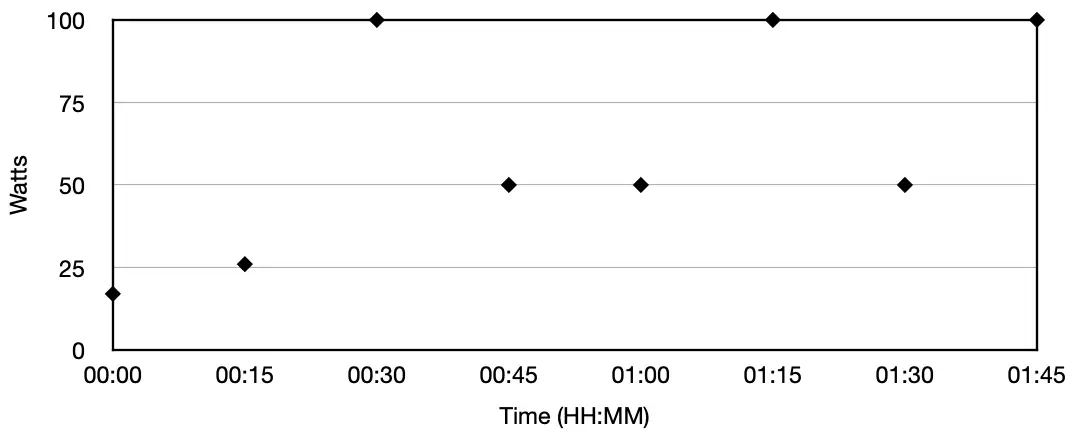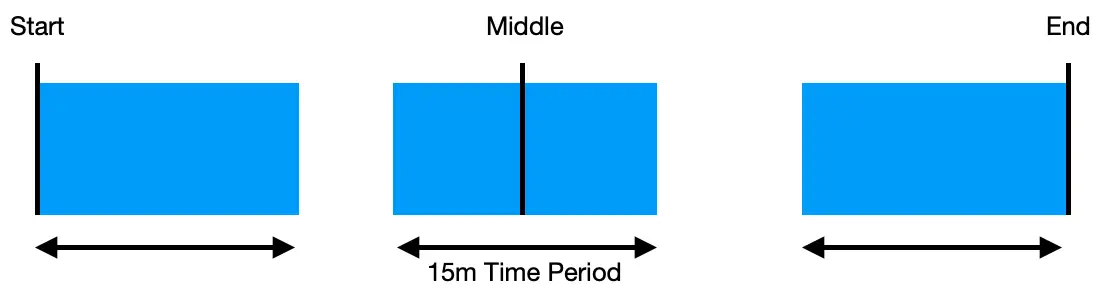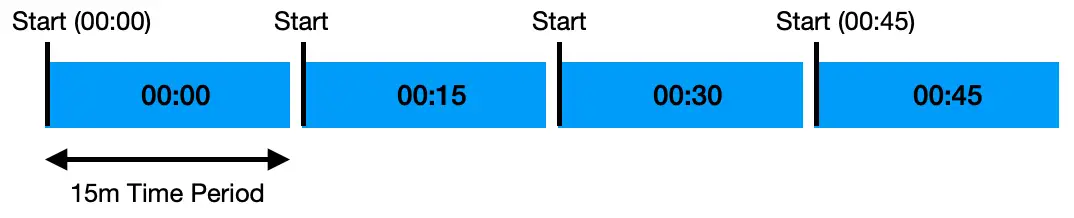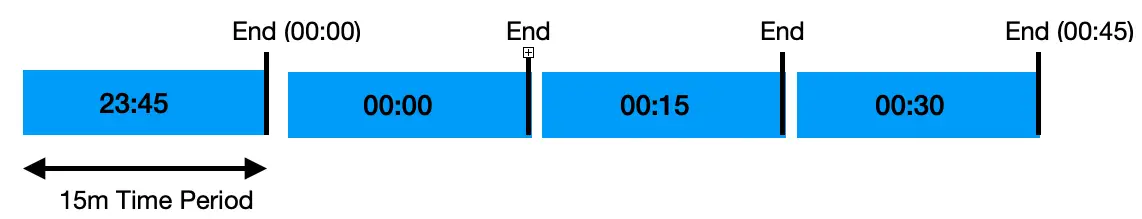# Calculating Electricity Usage From Instantaneous Data

How timestamps and windows need to be considered – If you’re gathering instantaneous time-series data such as electricity usage in Watts over specific time periods then, at some point, you’re going to want to convert this into usage data – i.e. kWh or suchlike.

### Sometimes this can be a little confusing, primarily due to a number of factors such as:

• The time period covered by the sample
• When the time series data was sampled related to the time period
• What unit of usage is required

Let’s address them all in turn.

## Time Periods

This is pretty straightforward and we can illustrate this with a simple example. Let’s imagine we’re monitoring the power consumed by a single electrical appliance on a circuit by putting in place a sensor that returns usage data, in Watts,  every 15m.

Every 15m we’ll receive a data point and this is what we’ll call our “time period”. Let’s plot it on a chart, not that we don’t join the lines as they’re instantaneous values as we have no information as to how to interpolate in any way between them.## Sample Period

It isn’t obvious at first, but it’s important to know what 15m time period outlined above the instantaneous sample points apply to - the start, the middle, or the end. This is best illustrated by a simple diagram,Remember, the instantaneous value isn’t being returned until the time period to which it applies is complete - so we’re not getting values for future events - it’s just what the sensor chooses as it’s default (or what it has been set to).

This may not seem immediately important, but it can have real implications later on when calculating usage. Anyway, let’s deal with how to calculate usage first, then look into how instantaneous sample time and time periods can affect calculations.

## Unit of Usage

So, if we’re getting instantaneous usage values every 15m (or 4 an hour) and we want to convert to kWh to display usage (and perhaps calculate a bill of some sort) just how do we do it?

### Let’s take a simple example and derive an equation from it:

If I receive 4 values (one every 15m for an hour), one of which are 100W. One time period is 100W for 15m (we assume usage is flat across that period as we have no other information about it) If this one time period were the only one for the hour and the rest were 0W then we’d have 100W for 1/4 of an hour and nothing for the rest.

So, we divide the value by 4 to get an average value for an hour (as there are 4 x 15m periods in an hour) which gives us Wh. To get kWh hours we divide by 1,000.

##### If we had receive 4 values, all of which were 100W,usage = ((100/4) + (100/4) + (100/4) + (100/4)) / 1,000 = 0.1 kWh.

Which, if you think about it makes perfect sense as in this case it’s effectively a continual usage of 100W for an hour, or 100 Wh, or 0.1 kWh.

## Subtleties

Why I mentioned where the sample was taken with respect to the time period applied to becomes relevant if we ask for an average usage over a period of time. Let’s say we ask for sample data between 00:00 and 00:59. If the sample data refers to the 15m time period following the sample, we’ll get this data:
(I’ve marked each blue block with the time period of the sample data for clarity).But what if the sensor had delivered instantaneous sample whereby the timestamp has referred to the end of each period?As can be seen, we may have inadvertently requested data that includes 15m of the previous hour and also misses the last 15m of this hour, simply because of the way the data is presented by the sensor.

It’s important to know to which window of time the instantaneous data applies.

### Note:

This becomes especially important when using Time Series databases, such a InfluxDB, where windowing calculations, such as aggregateWindow() (and timeSrc) in the Flux language have a parameter to specify to which window a timestamp applies when they generate aggregations.